# Electronics and Communication Engineering - Analog Electronics

31.

In figure the saturation collector current is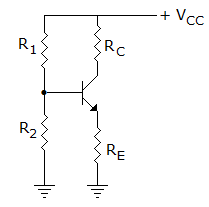A.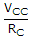B.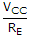C.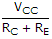D. none of the above

Explanation:

Since IE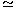IC,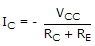.

32.

Consider the following statements : A clamper circuit

1. adds or subtracts a dc voltage to a waveform
2. does not change the waveform
3. amplifiers the waveform
Which are correct?

 A. 1, 2 B. 1, 3 C. 1, 2, 3 D. 2, 3

Explanation:

Clamper does not amplify the waveform. Hence 1 and 2 are correct.

33.

In figure v1 = 8 V and v2 = 8 V. Which diode will conduct?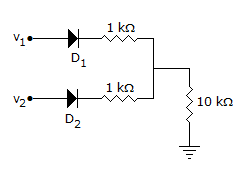A. D1 only B. D2 only C. Both D1 and D2 D. Neither D1 nor D2

Explanation:

Both D1 and D2 are forward biased.

34.

A forward voltage of 9 V is applied to a diode in series with a 1 kΩ load resistor. The voltage across load resistor is zero. It indicates that

 A. diode is short circuited B. diode is open circuited C. resistor is open circuited D. diode is either short circuited or open circuited

Explanation:

Since load voltage is zero, the current is zero and diode is open-circuited. If load was open-circuited, load voltage would be about 9 V.

35.

Which power amplifier can deliver maximum load power?

 A. Class A B. Class AB C. Class B D. Class C